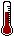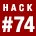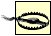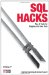# Hack 74. Compare and Synchronize TablesFind differences between tables with identical structures and similar data.

You might have a live database system, and a second database system mirror which you use for development and as a backup. Sometimes you might insert rows into your backup database for testing purposes. You want to check that the two versions of the database have not gotten out of sync with each other, and if they have, you want a minimum list of SQL commands to get them back to a synchronized state.

By way of an example, suppose you have two tables, original and backup. They have an identical schema, but different contents:

```CREATE TABLE original (
x VARCHAR(5) primary key,
y VARCHAR(20),
z INT
);
CREATE TABLE backup (
x VARCHAR(5) primary key,
y VARCHAR(20),
z INT
);
INSERT INTO original VALUES ('10','word1',100);
INSERT INTO original VALUES ('20','word2',200);
INSERT INTO original VALUES ('30','word3',300);
INSERT INTO backup SELECT * from original;

UPDATE original SET y='origword2' WHERE x='20';
UPDATE backup SET y='backword3' WHERE x='30';
INSERT INTO original VALUES ('40','word4',400);
INSERT INTO backup VALUES ('50','word5',500);
DELETE FROM original WHERE x='10';```

If you wanted to work out which rows were different between original and backup you could use standard SQL queries:

```SELECT CONCAT('INSERT INTO backup (x,y,z) VALUES (''
,o.x,'',''
,o.y,'',''
,o.z,'')') AS SQL
FROM original o LEFT OUTER JOIN backup b ON (o.x = b.x)
WHERE b.x IS NULL
UNION
SELECT CONCAT('DELETE FROM backup WHERE x='',b.x,''') AS SQL
FROM original o RIGHT OUTER JOIN backup b ON (o.x = b.x)
WHERE o.x IS NULL
UNION
SELECT CONCAT('UPDATE backup SET y=''
,o.y,'',set z=''
,o.z,'' WHERE x=''
,o.x,''') AS SQL
FROM original o INNER JOIN backup b ON (o.x = b.x)
WHERE o.y != b.y OR o.z != b.z
;```

The preceding SQL query produces the following for this example:

```+----------------------------------------------------------+
| SQL |
+----------------------------------------------------------+
| INSERT INTO backup (x,y,z) VALUES ('40','word4','400') |
| DELETE FROM backup WHERE x='10' |
| DELETE FROM backup WHERE x='50' |
| UPDATE backup SET y='origword2',set z='200' WHERE x='20' |
| UPDATE backup SET y='word3',set z='300' WHERE x='30' |
+----------------------------------------------------------+
5 rows in set (0.00 sec)```

The query is not perfect, because the UPDATE output always makes it appear that all nonkey columns need to be updated, even if the update would have been right with fewer SETs. Also, it requires three different queries, even though logically the analysis can be done in one pass. Lastly, it has to be specifically written for each comparison you want to make.

In Unix, you have the generalized diff command to check for file and directory differences. You can write a generalized program to perform a similar task for databases. The following code uses a single-pass algorithm to perform the comparison:

```#!/usr/bin/perl
use strict;
use DBI;

#====

sub testKey {
my (\$row,\$rrow,\$spos) = @_;
return 0 if (!defined \$row)&&(!defined \$rrow);
return -1 if (!defined \$rrow);
return 1 if (!defined \$row);
for(my \$i=\$spos; \$i<=\$#\$row; \$i++) {
return -1 if (\$row->[\$i] lt \$rrow->[\$i]);
return 1 if (\$row->[\$i] gt \$rrow->[\$i]);
}
return 0;
}
sub diff {
my (\$nonkeys,\$row,\$rrow) = @_;
my @set;
my @val;
for(my \$i=0; \$i<=\$#\$nonkeys; \$i++) {
next if (!defined \$row->[\$i])&&(!defined \$rrow->[\$i]);
if (!defined \$row->[\$i]) {push @set,\$nonkeys->[\$i]."=NULL";next;}
if ((!defined \$rrow->[\$i])||(\$row->[\$i] ne \$rrow->[\$i])) {
push @set,\$nonkeys->[\$i]."=?";
push @val,\$row->[\$i];
}
}
return (undef,@val) if \$#set<0;
return (join(",",@set),@val);
}
sub sync{
my (\$dbh,\$dbh2,\$table1,\$table2,\$keys,\$nonkeys) = @_;
my (\$found,\$missing,\$stale) = (0,0,0);
my @actions;
my \$sql = "SELECT ".join(",",@\$nonkeys,@\$keys)." FROM \$table1
ORDER BY ".join(",",@\$keys);
my \$sth = \$dbh->prepare(\$sql);
\$sth->execute( );
\$sql = "SELECT ".join(",",@\$nonkeys,@\$keys)." FROM \$table2
ORDER BY ".join(",",@\$keys);
my \$sth2 = \$dbh2->prepare(\$sql);
\$sth2->execute( );
my \$row = \$sth->fetchrow_arrayref( );
my \$rrow = \$sth2->fetchrow_arrayref( );
while ( \$row || \$rrow) {
my (\$getlocal,\$getremote) = (0,0);
my \$test = testKey(\$row,\$rrow,\$#\$nonkeys+1);
if (\$test == 0) {
# Keys Match : what about values
\$found++;
my (\$set,\$val) = diff(\$nonkeys,\$row,\$rrow);
if (defined \$set) {
my \$csql = "UPDATE \$table2 SET ".\$set;
\$csql.=" WHERE ".join("=? AND ",@\$keys)."=?";
my @k = @\$rrow; splice @k,0,\$#\$nonkeys+1;
push @actions,[\$csql,[@\$val,@k]];
}
(\$getlocal,\$getremote) = (1,1);
} elsif (\$test == -1) {
# Insert local row into remote
my \$csql =
"INSERT INTO \$table2 VALUES(".join(",",@\$nonkeys,@\$keys).") (";
\$csql .= '?,'x(\$#\$nonkeys+\$#\$keys+1)."?)";
push @actions,[\$csql,[@\$row]];
\$missing++;
\$getlocal++;
} else {
# Delete remote row
\$stale++;
my \$csql = "DELETE FROM \$table2 WHERE ";
\$csql.=join("=? AND ",@\$keys)."=?";
my @r = @\$rrow; splice @r,0,\$#\$nonkeys+1;
push @actions,[\$csql,@r];
\$getremote++;
}
\$row = \$sth->fetchrow_arrayref( ) if (\$getlocal);
\$rrow = \$sth2->fetchrow_arrayref( ) if (\$getremote);
}
print "Scan complete: \$found matches, \$missing missing. ",
"Records stale: \$stale
";
foreach my \$a (@actions) {
my \$out = \$a->;
foreach my \$v (@{\$a->}) { \$out =~ s/?/'\$v'/; }
print \$out."
";
}
}

# localdb,remotedb, tablename, [keys],[nonkeys]
sync(\$dbh1,\$dbh2, 'original','backup',[qw(x)], [qw(y z)]);```

This produces output similar to the following:

```[gordon@db book]\$ ./diff.pl
Scan complete: 2 matches, 1 missing. Records stale: 2
DELETE FROM backup WHERE x='10'
UPDATE backup SET y='origword2' WHERE x='20'
UPDATE backup SET y='word3' WHERE x='30'
INSERT INTO backup VALUES(y,z,x) ('word4','400','40')
DELETE FROM backup WHERE x='50'```

By using a single-pass approach, you obtain significant performance gains. The program does need to read both tables in their entirety once, but it linearly scans the tables in primary key order, and it needs only one row from each table to be in memory at any one time, so actually it runs quite efficiently. When you use this program in a situation where one table is on a local database and the other table is on a remote system, the one-pass code runs almost an order of magnitude faster than an implementation using the original three-pass query.None of the approaches shown here uses explicit locking. They are accurate provided no updates are occurring on the tables while the difference is being calculated. Even without locking, you will still find the data useful. You could run the diff and apply the changes without locking (thus producing and applying the majority of the differences), and then run the whole process again, with table locking, to finalize the sync.

You can extend the code to automatically apply the differences to the remote table. This would allow you to update your mirror on a regular schedule. Another practical application of this code would be to support multiple parallel database systems, each updated from a single master server. You could then write your application code to use one of the slave databases for the activities that don't require the most up-to-date information, but connect to the master database for more sensitive activities as well as for updating your database (and even use one of the slave databases as a backup if the master should ever fail). If you've ever wondered why some search engines give different results to users who are in different geographic locations, it may be that it's a massively distributed system with lazy updates!

9.11.1. Hacking the Hack

The code currently works best with keys which are strings. If your keys involve dates or numbers, the code could get confused. The testKey function is critical to the program working correctly. You need to replace lt and gt with < and > for numerical keys. Dates are much harder to handle. You could specify the type of each key as part of the function call, or have the program learn the types by guesswork, or analyze the schema of each table and determine the types directly from the database.

Date handling and schema processing are largely database-system dependent. To extend the routine to cover all interesting database systems would produce quite a lot of code, but you may want to extend this program yourself for your particular engine.SQL Hacks
ISBN: 0596527993
EAN: 2147483647
Year: 2004
Pages: 147